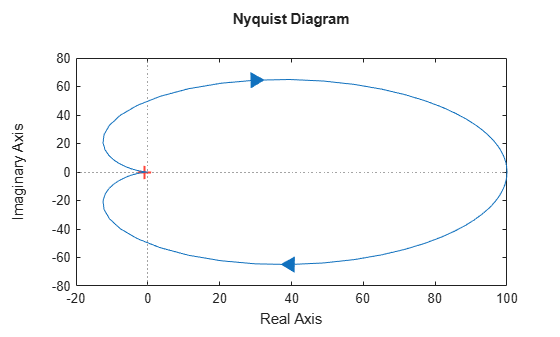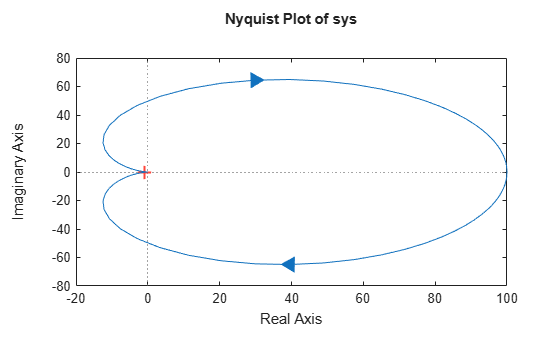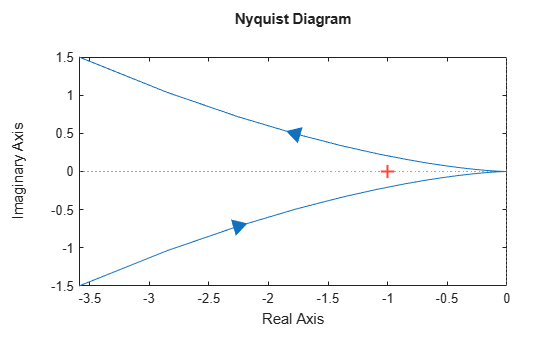Main Content

# nyquistplot

Nyquist plot with additional plot customization options

## Syntax

```h = nyquistplot(sys) nyquistplot(sys,{wmin,wmax}) nyquistplot(sys,w) nyquistplot(sys1,sys2,...,w) nyquistplot(AX,...) nyquistplot(..., plotoptions) ```

## Description

`h = nyquistplot(sys)` draws the Nyquist plot of the dynamic system model `sys`. It also returns the plot handle `h`. You can use this handle to customize the plot with the `getoptions` and `setoptions` commands. Type

```help nyquistoptions ```

for a list of available plot options.

The frequency range and number of points are chosen automatically. See `bode` for details on the notion of frequency in discrete time.

`nyquistplot(sys,{wmin,wmax})` draws the Nyquist plot for frequencies between `wmin` and `wmax` (in `rad/TimeUnit`, where `TimeUnit` is the time units of the input dynamic system, specified in the `TimeUnit` property of `sys`).

`nyquistplot(sys,w)` uses the user-supplied vector `w` of frequencies (in `rad/TimeUnit`, where `TimeUnit` is the time units of the input dynamic system, specified in the `TimeUnit` property of `sys`) at which the Nyquist response is to be evaluated. See `logspace` to generate logarithmically spaced frequency vectors.

`nyquistplot(sys1,sys2,...,w)` draws the Nyquist plots of multiple models `sys1`,`sys2`,... on a single plot. The frequency vector `w` is optional. You can also specify a color, line style, and marker for each system, as in

`nyquistplot(sys1,'r',sys2,'y--',sys3,'gx')`

`nyquistplot(AX,...)` plots into the axes with handle `AX`.

`nyquistplot(..., plotoptions)` plots the Nyquist response with the options specified in `plotoptions`. Type

```help nyquistoptions ```

for more details.

## Examples

### Customize Nyquist Plot Title

Create a Nyquist plot of a dynamic system model and store a handle to the plot.

```sys = tf(100,[1,2,1]); h = nyquistplot(sys);```Change the plot title to read "Nyquist Plot of sys." To do so, use `getoptions` to extract the existing plot options from the plot handle `h`.

`opt = getoptions(h)`
```opt = FreqUnits: 'rad/s' MagUnits: 'dB' PhaseUnits: 'deg' ShowFullContour: 'on' ConfidenceRegionNumberSD: 1 ConfidenceRegionDisplaySpacing: 5 IOGrouping: 'none' InputLabels: [1x1 struct] OutputLabels: [1x1 struct] InputVisible: {'on'} OutputVisible: {'on'} Title: [1x1 struct] XLabel: [1x1 struct] YLabel: [1x1 struct] TickLabel: [1x1 struct] Grid: 'off' GridColor: [0.1500 0.1500 0.1500] XLim: {[-20 100]} YLim: {[-80 80]} XLimMode: {'auto'} YLimMode: {'auto'} ```

The `Title` option is a structure with several fields.

`opt.Title`
```ans = struct with fields: String: 'Nyquist Diagram' FontSize: 11 FontWeight: 'bold' FontAngle: 'normal' Color: [0 0 0] Interpreter: 'tex' ```

Change the `String` field of the `Title` structure, and use `setoptions` to apply the change to the plot.

```opt.Title.String = 'Nyquist Plot of sys'; setoptions(h,opt)```### Zoom on Critical Point

Plot the Nyquist frequency response of a dynamic system. Assign a variable name to the plot handle so that you can access it for further manipulation.

```sys = tf(100,[1,2,1]); h = nyquistplot(sys);```Zoom in on the critical point, (–1,0). You can do so interactively by right-clicking on the plot and selecting Zoom on (-1,0). Alternatively, use the `zoomcp` command on the plot handle `h`.

`zoomcp(h)`### Plot Identified Models with Confidence Regions at Selected Points

Compare the frequency responses of identified state-space models of order 2 and 6 along with their `1-std` confidence regions rendered at every 50th frequency sample.

```load iddata1 sys1 = n4sid(z1,2); % discrete-time IDSS model of order 2 sys2 = n4sid(z1,6); % discrete-time IDSS model of order 6```

Both models produce about 76% fit to data. However, `sys2` shows higher uncertainty in its frequency response, especially close to Nyquist frequency as shown by the plot. To see this, generate a Nyquist plot and show the confidence region at a subset of the points at which the Nyquist response is displayed.

```w = linspace(10,10*pi,256); h = nyquistplot(sys1,sys2,w); setoptions(h,'ConfidenceRegionDisplaySpacing',50,... 'ShowFullContour','off');```

To turn on the confidence region display, right-click the plot and select Characteristics > Confidence Region.## Tips

• You can change the properties of your plot, for example the units. For information on the ways to change properties of your plots, see Ways to Customize Plots (Control System Toolbox).

• There are two zoom options available from the right-click menu that apply specifically to Nyquist plots:

• Full View — Clips unbounded branches of the Nyquist plot, but still includes the critical point (–1, 0).

• Zoom on (-1,0) — Zooms around the critical point (–1,0). To access critical-point zoom programmatically, use the `zoomcp` command. See Zoom on Critical Point.

• To activate data markers that display the real and imaginary values at a given frequency, click anywhere on the curve. The following figure shows a `nyquist` plot with a data marker.## See Also

Introduced in R2012a

## SupportGet trial now